# KSEEB Solutions for Class 8 Maths Chapter 11 Congruency of Triangles Additional Questions

Students can Download Maths Chapter 11 Congruency of Triangles Additional Questions and Answers, Notes Pdf, KSEEB Solutions for Class 8 Maths helps you to revise the complete Karnataka State Board Syllabus and score more marks in your examinations.

## Karnataka State Syllabus Class 8 Maths Chapter 11 Congruency of Triangles Additional Questions

Question 1.
Fill in the blanks to make the statements true
a. In right triangle the hypotenuse is the greatest side
b. The sum of three altitudes of a triangle is less than its perimeter.
c. The sum of any two sides of a triangle is greater than the third side.
d. If two angles of a triangle are unequal, then the smaller angle has the smaller side opposite to it.
e. If two sides of a triangle are unequal, then the larger side has larger angle opposite to it.Question 2.
Justify the following statements with reasons
a. The sum of three sides of triangles is more than the sum of its altitudes
Solution:
In ∆ ABC Let AD, BE and CF be the altitudes The perpendicular is the shortest distance from a point to B the line∴ AD < AB, BE < BC and CF < AC
∴ AD + BE + CF < AB T BC + AC or ∴ AB + BC + AC > AD + BE + CF

b. The sum of any two sides of a triangle is greater than twice the median drawn to the third side.
Solution:
In ∆ ABC AP is the median from A to BCTo prove : AB + AC > 2APQ Construction : Produce AP to Q such that AP = PQ join CQ
Proof: In ∆ APB and ∆ PQG
BP = PC [P is the midpoint of BC]
AP = PQ [construction]
∠APB = ∠CPQ [V.O.A]
∴ ∆ APB = ∆ CPQ [SAS postulate]
∴ AB = CQ [corresponding sides]
In ∆ ACQ, AC + CQ > AQ [Sum of two sides is greater than the third side]
AC + AB > AP + PQ
AC + AB > 2 AP
[∴ AB = CQ and AP = PQ]c. Difference of any two sides is less than the third side
Solution:
In ∆ ABC
To prove (i) AC – AB < BC (ii) BC – AC < AB (iii) BC – AB < AC Construction Let AC > AB . On AC mark a point D such that AB = AD. Join BD
∴∠1 = ∠2 [Base angles of an isosceles triangle]
Extr ∠2 = ∠4 + ∠3
∠2 > ∠4 [Exterior angle > each interior opp angle]
∴ Extr ∠3 = ∠1 + ∠A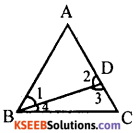∠3 > ∠1 [Exterior angle > each interior angle]
∠3 >∠2 [∵ ∠1 = ∠2]
∴ ∠3 > ∠2 and ∠2 > ∠4 means ∠3 > ∠4
∴ BC > DC [side opposite to larger angle is larger].
BC >AC – AB [∵ AD = AB]
or
AC – AB < BC
Similarly BC – AC < AB and BC – AB < ACQuestion 3.
Two triangles ABC and DBC have common base BC. Suppose AB = DC and ∠ABC – ∠BCD prove that AC = BD.
Solution: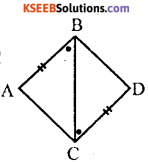In ∆ ABC and ∆ DBC AB = DC (data) ∠ABC = ∠BCD [Data]
BC = BC (common side)
∴ ∆ ABC ≅ ∆ DBC (SAS postulate)
∴ AC = BD (Corresponding sides)

Question 4.
Let AB and CD be two line segments such that AD and BC intersect at O. Suppose AO = OC and BO = OD. Prove that AB = CD.
Solution:In ∆ AOB and ∆ COD
AO = OC [data]
BO = OD [data]
∠AOB = ∠COD [vertically oppsoite angles]
∴ ∆ AOB ≅ ∆ COD [SAS postulate]
∴ AB = CD [corresponding sides]Question 5.
Let ABC be a triangle. Draw a triangle BDC externally on BC such that AB = BD and AC = CD. Prove that ∆ ABC ≅ ∆ DBC
Solution: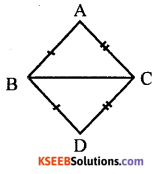In ∆ ABC and ∆ DBC
AB = BD [data]
AC = CD [data]
BC = BC [common side]
∴ ∆ ABC = ADBC [SSS postulate]

Question 6.
Let ABCD be a square and let points P on AB and Q on DC be such that DP = AQ. Prove that BP = CQ.
Solution:In ∆ APD and ∆ AQD ∠PAD = ∠QDA [= 90°]
[common side]
DP = AQ [data]
∆ APD ≅ ∆ AQD [RHS postulate]
∴ AP = DQ [corresponding sides]
∴ AB = DC [siodes of a square]
AB – AP = DC – DQ
BP = CQ [Axiom 3]Question 7.
In a triangle ABC, AB = AC. Suppose P is a point on AB and Q is a point on AC such that AP = AQ. Prove that ∆ APC ≅ ∆ AQB.
Solution: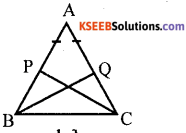In ∆ APC and ∆ AQB AP = AQ [data]
AC = AB [data]
∠PAC – ∠BAQ [common angle]
∴ ∆ APC ≅ ∆ AQB [SAS postulate]

Question 8.
In an isosceles triangle, if the vertex angle is twice the sum of the base angles, calculate the angles of the triangle.
Solution: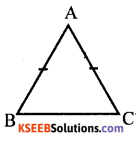In ∆ ABC, Let AB = BC A
∠B = ∠C [∴ base angles of isosceles triangle]
∠A = 2[∠B + ∠C] [data] B C
∠A = 2[∠B + ∠B] [∴ ∠B = ∠C]
∠A = 2[2∠B]
∠A = 4∠B
In ∆ ABC, ∠A + ∠B + ∠C = 180° [Sum of the angles of the triangle]
4∠B + ∠B = ∠B = 180° [substituting for ∠A = 4∠B and |C = [B]
6∠B = 180° ∠B = $$\frac{180}{6}$$ = 30°
∠B = 30° ∠C = 30°
∠A = 4∠B = 4 x 30 = 120°Question 9.
If the bisector of the vertical angle of a triangle bisects the base, show that the triangle is isosceles.
Solution:Data : In ∆ ABC, AD bisects ∠A and BD = DC
To prove : AB = AC Construction : produce AD to P such that AD = DP and B joint PC Proof : In
BD = DC [data]
∠APB = ∠CDP [V.O.A]
∴ ∆ ABD ≅ ∆ CDP [SAS postulate]
∴ AB = CP [corresponding sides]
∴ CP = AC
[sides opposite to equal angles] But CP = AB [proved]
∴ AB = AC

Question 10.
Suppose ABC is an isosceles triangle with AB = AC side BA is produced to D such that BA = AD. Prove that ∠BCD is a right angle .
Solution: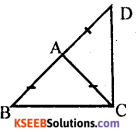Let ∠ABC = x
In ∆ ABC, AB = AC [data]
∴ ∠ABC = ∠ACB = x [Base angles of isosceles triangle]
AB = AC = AD [data]
[Base angles of isosceles triangle]
In ∆ BCD, ∠DBC +∠BCD + ∠BDC = 180°
[Sum of the angles of a triangle]
∠DBC + ∠ACD + ∠ACB + ∠BDC = 180°
x + y + x + y = 180°
2x + 2y = 180°
2(x + y) = 180°
x + y = $$\frac{180}{2}$$ =90°
∠BCD = 90° [ ∵ x + y = ∠BCDQuestion 11.
Let AB, CD be two line segements such that AB || CD and AD || BC. Let E be the midpoint of BC and let DE extended meet AB in F. Prove that AB = BF.
Solution:
In ∆ BEF and ∆ DEC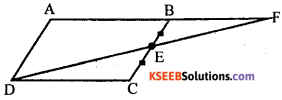BE = EC [data]
∠BEF – ∠DEC [vertically opposite angles]
∠EBF = ∠ECF [Alternate angles]
∴ ∆ BEF ≅ ∆ DEC [ASA postulate]
∴ BF = DC [corresponding sides]
But AB = DC [data]
∴ AB = BFerror: Content is protected !!